Thiago Martins
Vespa Data Scientist

# Build a basic text search application from python with Vespa: Part 2

Evaluate search engine experiments using Python.

We want to enable Vespa users to run their experiments from python. This tutorial illustrates how to define query models and evaluation metrics to perform search engine experiments.

UPDATE 2023-02-13: Code examples and links are updated to work with the latest releases of pyvespa and learntorank.Photo by Eugene Golovesov on Unsplash

We show how to use the pyvespa API to run search engine experiments based on the text search app we built in the first part of this tutorial series. Specifically, we compare two different matching operators and show how to reduce the number of documents matched by the queries while keeping similar recall and precision metrics.

We assume that you have followed the first tutorial and have a variable `app` holding the `Vespa` connection instance that we established there. This connection should be pointing to a Docker container named `cord19` running the Vespa application.

We will continue to use the CORD19 sample data that fed the search app in the first tutorial. In addition, we are going to feed a few additional data points to make it possible to get relevant metrics from our experiments. We tried to minimize the amount of data required to make this tutorial easy to reproduce. You can download the additional 494 data points below:

``````from pandas import read_csv

``````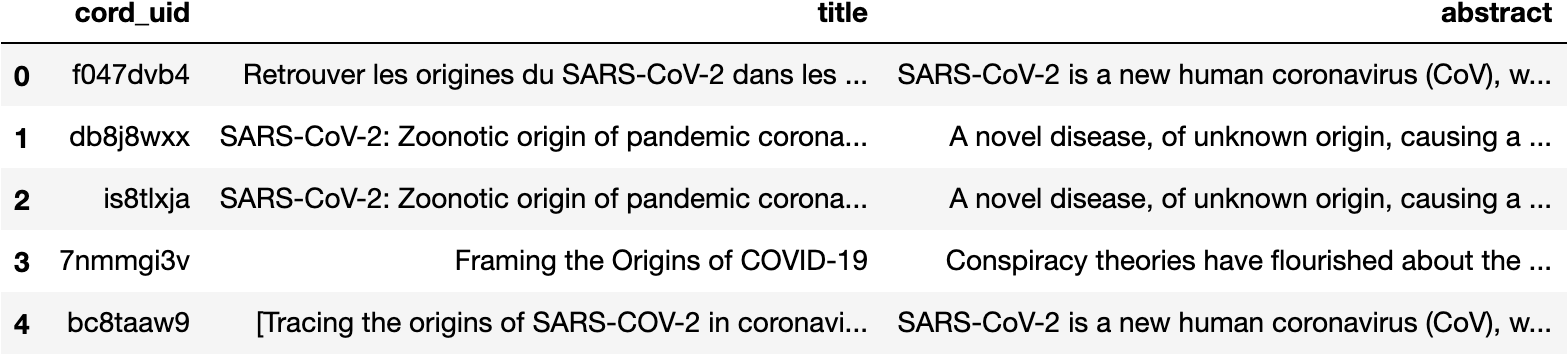We can then feed the data we just downloaded to the `app` via the `feed_data_point` method:

``````for idx, row in parsed_feed.iterrows():
fields = {
"cord_uid": str(row["cord_uid"]),
"title": str(row["title"]),
"abstract": str(row["abstract"])
}
response = app.feed_data_point(
schema = "cord19",
data_id = str(row["cord_uid"]),
fields = fields,
)
``````

## Define query models to compare

A `QueryModel` is an abstraction that encapsulates all the relevant information controlling how your app matches and ranks documents. Since we are dealing with a simple text search app here, we will start by creating two query models that use BM25 to rank but differ on how they match documents.

``````from learntorank.query import QueryModel, OR, WeakAnd, Ranking

or_bm25 = QueryModel(
name="or_bm25",
match_phase=OR(),
ranking=Ranking(name="bm25")
)
``````

The first model is named `or_bm25` and will match all the documents that share at least one token with the query.

``````from learntorank.query import WeakAnd

wand_bm25 = QueryModel(
name="wand_bm25",
match_phase=WeakAnd(hits=10),
ranking=Ranking(name="bm25")
)
``````

The second model is named `wand_bm25` and uses the `WeakAnd` operator, considered an accelerated OR operator. The next section shows that the `WeakAnd` operator matches fewer documents without affecting the recall and precision metrics for the case considered here. We also analyze the optimal `hits` parameter to use for our specific application.

## Run experiments

We can define which metrics we want to compute when running our experiments.

``````from learntorank.evaluation import MatchRatio, Recall, NormalizedDiscountedCumulativeGain

eval_metrics = [
MatchRatio(),
Recall(at=10),
NormalizedDiscountedCumulativeGain(at=10)
]
``````

`MatchRatio` computes the fraction of the document corpus matched by the queries. This metric will be critical when comparing match phase operators such as the `OR` and the `WeakAnd`. In addition, we compute Recall and NDCG metrics.

We can download labeled data to perform our experiments and compare query models. In our sample data, we have 50 queries, and each has a relevant document associated with them.

``````import json, requests

requests.get("https://data.vespa.oath.cloud/blog/cord19/labeled_data.json").text
)
labeled_data[:3]
``````
``````[{'query_id': 1,
'relevant_docs': [{'id': 'kqqantwg', 'score': 2}],
'query': 'coronavirus origin'},
{'query_id': 2,
'relevant_docs': [{'id': '526elsrf', 'score': 2}],
'query': 'coronavirus response to weather changes'},
{'query_id': 3,
'relevant_docs': [{'id': '5jl6ltfj', 'score': 1}],
'query': 'coronavirus immunity'}]
``````

### Evaluate

Once we have labeled data, the evaluation metrics to compute, and the query models we want to compare, we can run experiments with the `evaluate` method. The `cord_uid` field of the Vespa application should match the `id` of the relevant documents.

``````from learntorank.evaluation import evaluate

evaluation = evaluate(
app=app,
labeled_data=labeled_data,
query_model=[or_bm25, wand_bm25],
eval_metrics=eval_metrics,
id_field="cord_uid",
)
evaluation
``````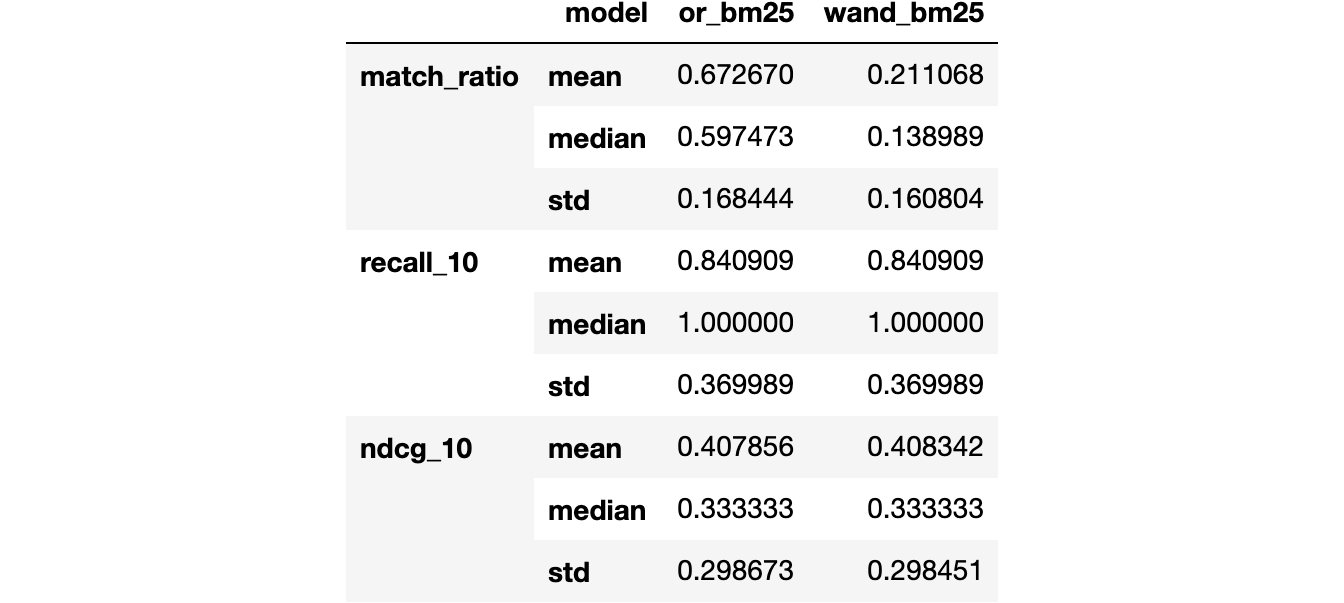The result shows that, on average, we match 67% of our document corpus when using the `OR` operator and 21% when using the `WeakAnd` operator. The reduction in matched documents did not affect the recall and the NDCG metrics, which stayed at around 0.84 and 0.40, respectively. The Match Ratio will get even better when we experiment with the `hits` parameter of the `WeakAnd` further down in this tutorial.

There are different options available to configure the output of the `evaluate` method.

### Specify summary statistics

The `evaluate` method returns the mean, the median, and the standard deviation of the metrics by default. We can customize this by specifying the desired `aggregators`. Below we choose the mean, the max, and the min as an example.

``````evaluation = evaluate(
app=app,
labeled_data=labeled_data,
query_model=[or_bm25, wand_bm25],
eval_metrics=eval_metrics,
id_field="cord_uid",
aggregators=["mean", "min", "max"]
)
evaluation
``````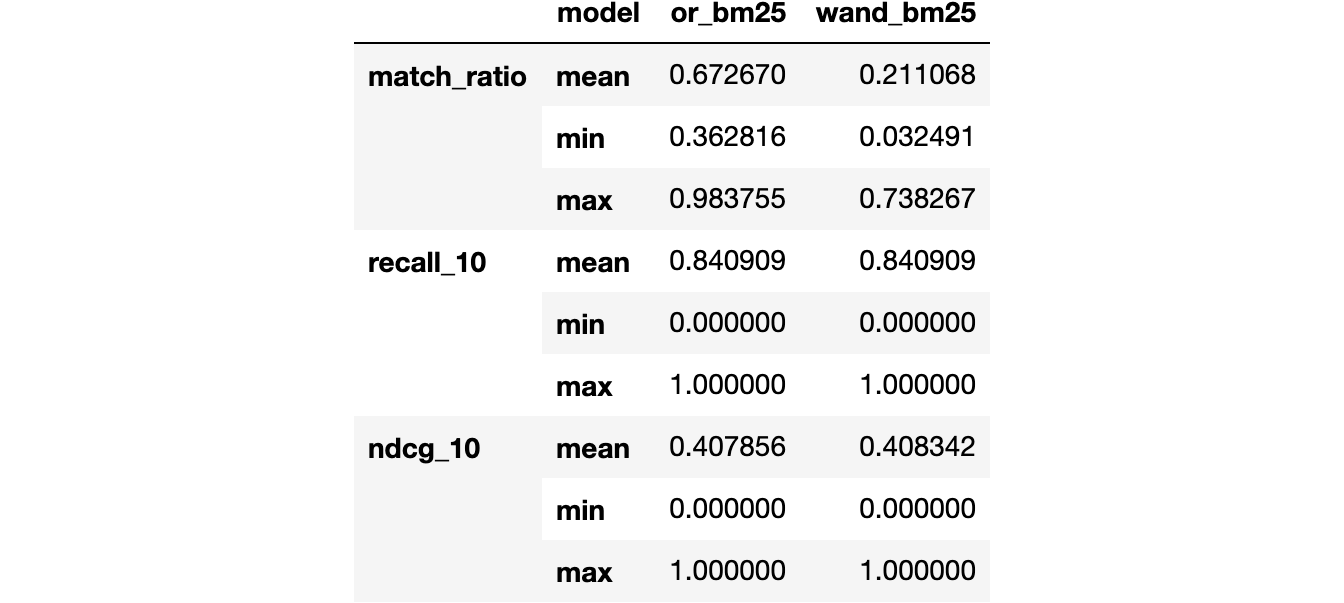### Check detailed metrics output

Some of the metrics have intermediate results that might be of interest. For example, the `MatchRatio` metric requires us to compute the number of matched documents (retrieved_docs) and the number of documents available to be retrieved (docs_available). We can output those intermediate steps by setting `detailed_metrics=True`.

``````evaluation = evaluate(
app=app,
labeled_data=labeled_data,
query_model=[or_bm25, wand_bm25],
eval_metrics=eval_metrics,
id_field="cord_uid",
aggregators=["mean"],
detailed_metrics=True
)
evaluation
``````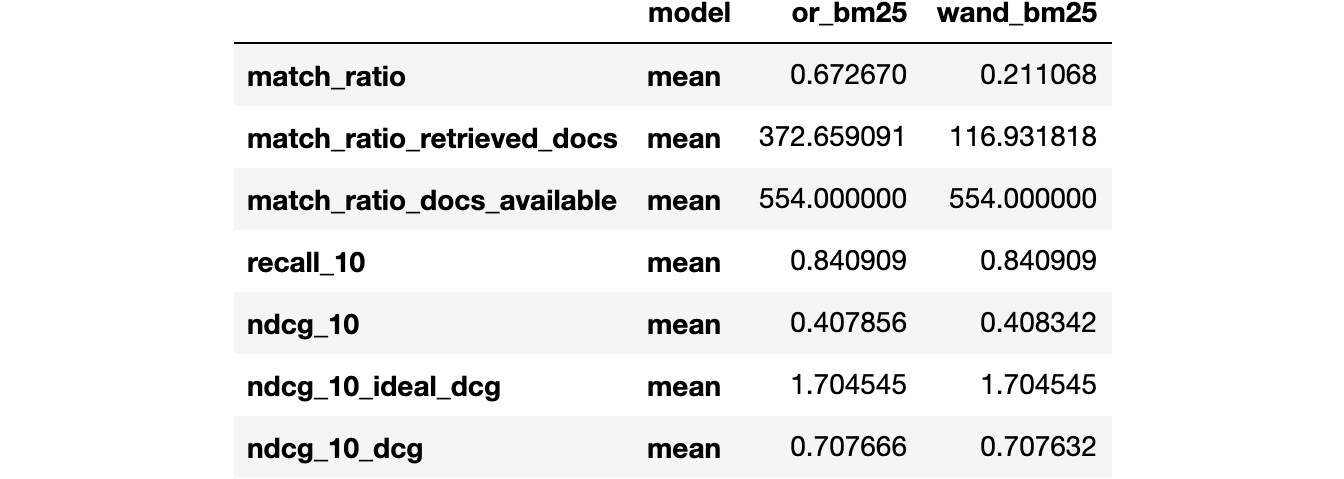### Get per-query results

When debugging the results, it is often helpful to look at the metrics on a per-query basis, which is available by setting `per_query=True.`

``````evaluation = evaluate(
app=app,
labeled_data=labeled_data,
query_model=[or_bm25, wand_bm25],
eval_metrics=eval_metrics,
id_field="cord_uid",
per_query=True
)
``````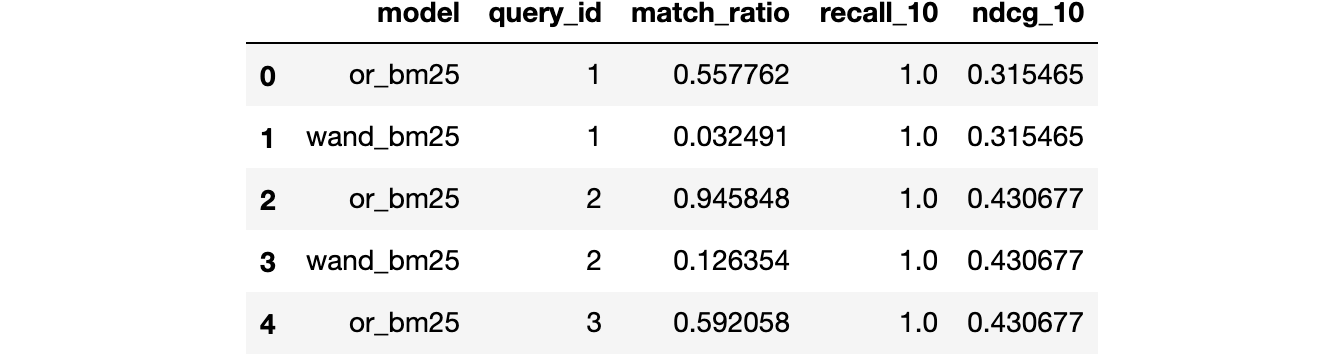## Find optimal WeakAnd parameter

We can use the same evaluation framework to find the optimal `hits` parameter of the `WeakAnd` operator for this specific application. To do that, we can define a list of query models that only differ by the `hits` parameter.

``````wand_models = [QueryModel(
name="wand_{}_bm25".format(hits),
match_phase=WeakAnd(hits=hits),
ranking=Ranking(name="bm25")
) for hits in range(1, 11)]
``````

We can then call `evaluate` as before and show the match ratio and recall for each of the options defined above.

``````evaluation = evaluate(
app=app,
labeled_data=labeled_data,
query_model=wand_models,
eval_metrics=eval_metrics,
id_field="cord_uid",
aggregators=["mean"],
)
evaluation.loc[["match_ratio", "recall_10"], ["wand_{}_bm25".format(hits) for hits in range(1, 11)]]
``````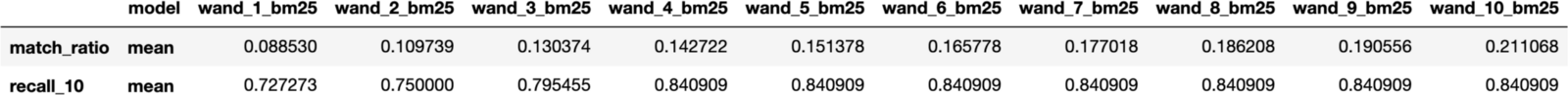As expected, we can see that a higher `hits` parameter implies a higher match ratio. But the recall metric remains the same as long as we pick `hits > 3`. So, using `WeakAnd` with `hits = 4` is enough for this specific application and dataset, leading to a further reduction in the number of documents matched on average by our queries.

Clean up:

``````vespa_docker.container.stop()
vespa_docker.container.remove()
``````

## Conclusion

We want to enable Vespa users to run their experiments from python. This tutorial illustrates how to define query models and evaluation metrics to run search engine experiments via the evaluate method. We used a simple example that compares two different match operators and another that optimizes the parameter of one of those operators. Our key finding is that we can reduce the size of the retrieved set of hits without losing recall and precision by using the `WeakAnd` instead of the `OR` match operator.

The following Vespa resources are related to the topics explored by the experiments presented here: